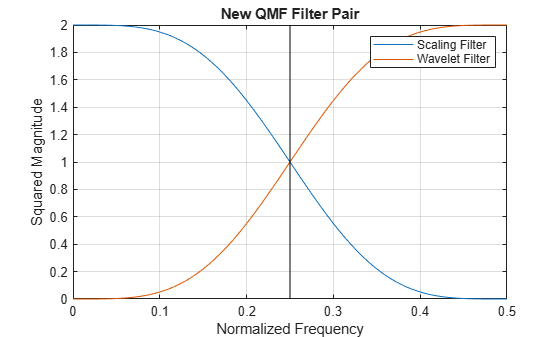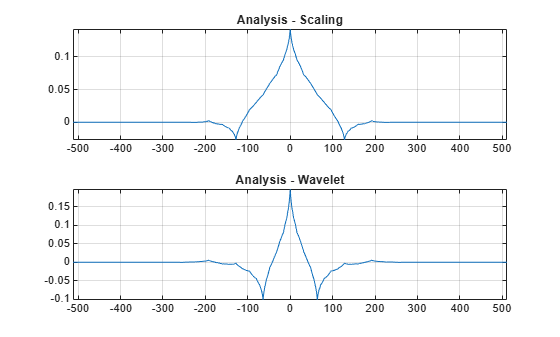This example shows how to add an orthogonal quadrature mirror filter (QMF) pair and biorthogonal wavelet filter quadruple to Wavelet Toolbox™. While Wavelet Toolbox™ already contains many of the most widely used orthogonal and biorthogonal wavelet families, including the Daubechies' extremal-phase, the Daubechies' least-asymmetric phase, the coiflet, the Fejér-Korovkin filters, and biorthogonal spline wavelets, you can easily add your own filters and use the filter in any of the discrete wavelet or wavelet packet algorithms.

This example adds the Beylkin(18) QMF filter pair to the toolbox and shows how to subsequently use the filter in discrete wavelet analysis. The example then demonstrates how to verify the necessary and sufficient conditions for the QMF pair to constitute a scaling and wavelet filter. After the adding the QMF pair, the example adds the nearly-orthogonal biorthogonal wavelet quadruple based on the Laplacian pyramid scheme of Burt and Adelson (Table 8.4 on page 283 in ).

First, you must have some way of obtaining the coefficients. In this case, here are the coefficients for the lowpass (scaling) Beylkin(18) filter. You only need a valid scaling filter. The `wfilters` function creates the corresponding wavelet filter for you.

```beyl = [9.93057653743539270E-02 4.24215360812961410E-01 6.99825214056600590E-01 4.49718251149468670E-01 -1.10927598348234300E-01 -2.64497231446384820E-01 2.69003088036903200E-02 1.55538731877093800E-01 -1.75207462665296490E-02 -8.85436306229248350E-02 1.96798660443221200E-02 4.29163872741922730E-02 -1.74604086960288290E-02 -1.43658079688526110E-02 1.00404118446319900E-02 1.48423478247234610E-03 -2.73603162625860610E-03 6.40485328521245350E-04];```

Save the Beylkin(18) filter and add the new filter to the toolbox. To add to the toolbox an orthogonal wavelet that is defined in a MAT-file, the name of the MAT-file and the variable containing the filter coefficients must match. The name must be less than five characters long.

`save beyl beyl`

Use `wavemngr` to add the wavelet filter to the toolbox. Define the wavelet family name and the short name used to access the filter. The short name must be the same name as the MAT-file. Define the wavelet type to be 1. Type 1 wavelets are orthogonal wavelets in the toolbox. Because you are adding only one wavelet in this family, define the NUMS variable input to `wavemngr` to be an empty string.

```familyName = 'beylkin'; familyShortName = 'beyl'; familyWaveType = 1; familyNums = ''; fileWaveName = 'beyl.mat';```

Add the wavelet using `wavemngr`.

`wavemngr('add',familyName,familyShortName,familyWaveType,familyNums,fileWaveName)`

Verify that the wavelet has been added to the toolbox.

`wavemngr('read')`
```ans = 19x35 char array '===================================' 'Haar ->->haar ' 'Daubechies ->->db ' 'Symlets ->->sym ' 'Coiflets ->->coif ' 'BiorSplines ->->bior ' 'ReverseBior ->->rbio ' 'Meyer ->->meyr ' 'DMeyer ->->dmey ' 'Gaussian ->->gaus ' 'Mexican_hat ->->mexh ' 'Morlet ->->morl ' 'Complex Gaussian ->->cgau ' 'Shannon ->->shan ' 'Frequency B-Spline->->fbsp ' 'Complex Morlet ->->cmor ' 'Fejer-Korovkin ->->fk ' 'beylkin ->->beyl ' '===================================' ```

You can now use the wavelet to analyze signals or images. For example, load an ECG signal and obtain the MODWT of the signal down to level four using the Beylkin(18) filter.

```load wecg wtecg = modwt(wecg,'beyl',4);```

Load a box image, obtain the 2-D DWT using the Beylkin(18) filter. Show the level-one diagonal detail coefficients.

```load xbox [C,S] = wavedec2(xbox,1,'beyl'); [H,V,D] = detcoef2('all',C,S,1); subplot(2,1,1) imagesc(xbox) axis off title('Original Image') subplot(2,1,2) imagesc(D) axis off title('Level-One Diagonal Coefficients')```Finally, verify that the new filter satisfies the conditions for an orthogonal QMF pair. Obtain the scaling (lowpass) and wavelet (highpass) filters.

`[Lo,Hi] = wfilters('beyl');`

Sum the lowpass filter coefficients to verify that the sum equals $\sqrt{2}$. Sum the wavelet filter coefficients and verify that the sum is 0.

`sum(Lo)`
```ans = 1.4142 ```
`sum(Hi)`
```ans = -1.9873e-16 ```

Verify that the autocorrelation of the scaling and wavelet filters at all even nonzero lags is 0.

```[Clow,lags] = xcorr(Lo,Lo,10); Chigh = xcorr(Hi,Hi,10); subplot(2,1,1) stem(lags,Clow,'markerfacecolor',[0 0 1]) grid on title('Autocorrelation of Scaling Filter') subplot(2,1,2) stem(lags,Chigh,'markerfacecolor',[0 0 1]) grid on title('Autocorrelation of Wavelet Filter')```Note that the autocorrelation values in both plots is zero for nonzero even lags. Show that the cross-correlation of the scaling and wavelet filter is zero at all even lags.

```[xcr,lags] = xcorr(Lo,Hi,10); figure stem(lags,xcr,'markerfacecolor',[0 0 1]) title('Cross-correlation of QMF filters')```The final criterion states the sum of squared magnitudes of the Fourier transforms of scaling and wavelet filters at each frequency is equal to 2. In other words, let $G\left(f\right)$ be the Fourier transform of the scaling filter and $H\left(f\right)$ be the Fourier transform of the wavelet filter. The following holds for all $f$: $|H\left(f\right){|}^{2}+|G\left(f\right){|}^{2}=2$. The DFT version of this equality is: $|{G}_{{2}^{m}kmodN}{|}^{2}+|{H}_{{2}^{m}kmodN}{|}^{2}=2$ for any $m$. Check this for the Beylkin(18) filter with $m=0$.

```N = numel(Lo); LoDFT = fft(Lo); HiDFT = fft(Hi); k = 0:N-1; m = 0; sumDFTmags = abs(LoDFT(1+mod(2^m*k,N))).^2+abs(HiDFT(1+mod(2^m*k,N))).^2```
```sumDFTmags = 18×1 2.0000 2.0000 2.0000 2.0000 2.0000 2.0000 2.0000 2.0000 2.0000 2.0000 ⋮ ```

All the values are equal to 2 as expected. To understand why these filters are called quadrature mirror filters, visualize the squared-magnitude frequency responses of the scaling and wavelet filters.

```nfft = 512; F = 0:1/nfft:1/2; LoDFT = fft(Lo,nfft); HiDFT = fft(Hi,nfft); figure plot(F,abs(LoDFT(1:nfft/2+1)).^2,'DisplayName','Scaling Filter') hold on plot(F,abs(HiDFT(1:nfft/2+1)).^2,'r','DisplayName','Wavelet Filter') xlabel('Frequency') ylabel('Squared Magnitude') title('Beylkin(18) QMF Filter Pair') grid on plot([1/4 1/4], [0 2],'k','HandleVisibility','off') legend show```Note the magnitude responses are symmetric, or mirror images, of each other around the quadrature frequency of 1/4.

The following code removes the Beylkin(18) wavelet filter.

```wavemngr('del',familyShortName); delete('beyl.mat')```

Adding a biorthogonal wavelet to the toolbox is similar to adding a QMF. You provide valid lowpass (scaling) filters pair used in analysis and synthesis. The `wfilters` function will generate the highpass filters.

To be recognized by `wfilters`, the analysis scaling filter must be assigned to the variable `Df`, and the synthesis scaling filter must be assigned to the variable `Rf`. The biorthogonal scaling filters do not have to be of even equal length. The output biorthogonal filter pairs created will have even equal lengths. Here are the scaling function pairs of the nearly-orthogonal biorthogonal wavelet quadruple based on the Laplacian pyramid scheme of Burt and Adelson.

```Df = [-1 5 12 5 -1]/20*sqrt(2); Rf = [-3 -15 73 170 73 -15 -3]/280*sqrt(2);```

Save the filters to a MAT-file.

`save burt Df Rf`

Use `wavemngr` to add the biorthogonal wavelet filters to the toolbox. Define the wavelet family name and the short name used to access the filter. The short name must match the name of the MAT-file. Since the wavelets are biorthogonal, set the wavelet type to be 2. Because you are adding only one wavelet in this family, define the NUMS variable input to `wavemngr` to be an empty string.

```familyName = 'burtAdelson'; familyShortName = 'burt'; familyWaveType = 2; familyNums = ''; fileWaveName = 'burt.mat'; wavemngr('add',familyName,familyShortName,familyWaveType,familyNums,fileWaveName)```

Verify that the biorthogonal wavelet has been added to the toolbox.

`wavemngr('read')`
```ans = 19x35 char array '===================================' 'Haar ->->haar ' 'Daubechies ->->db ' 'Symlets ->->sym ' 'Coiflets ->->coif ' 'BiorSplines ->->bior ' 'ReverseBior ->->rbio ' 'Meyer ->->meyr ' 'DMeyer ->->dmey ' 'Gaussian ->->gaus ' 'Mexican_hat ->->mexh ' 'Morlet ->->morl ' 'Complex Gaussian ->->cgau ' 'Shannon ->->shan ' 'Frequency B-Spline->->fbsp ' 'Complex Morlet ->->cmor ' 'Fejer-Korovkin ->->fk ' 'burtAdelson ->->burt ' '===================================' ```

You can now use the wavelet within the toolbox. Create an analysis DWT filter bank using the `burt` wavelet. Confirm the DWT filter bank is biorthogonal. Plot the magnitude frequency responses of the wavelet bandpass filters and coarsest resolution scaling function.

```fb = dwtfilterbank('Wavelet','burt'); isBiorthogonal(fb)```
```ans = logical 1 ```
`freqz(fb)`Obtain the wavelet and scaling functions of the filter bank. Plot the wavelet and scaling functions at the coarsest scale.

```[fb_phi,t] = scalingfunctions(fb); [fb_psi,~] = wavelets(fb); subplot(2,1,1) plot(t,fb_phi(end,:)) axis tight grid on title('Analysis - Scaling') subplot(2,1,2) plot(t,fb_psi(end,:)) axis tight grid on title('Analysis - Wavelet')```Create a synthesis DWT filter bank using the `burt` wavelet. Compute the framebounds.

```fb2 = dwtfilterbank('Wavelet','burt','FilterType','Synthesis','Level',4); [synthesisLowerBound,synthesisUpperBound] = framebounds(fb2)```
```synthesisLowerBound = 0.9800 ```
```synthesisUpperBound = 1.0509 ```

Obtain the lowpass and highpass analysis and synthesis filters associated with `burt`. Note the output filters are all of equal even length. Confirm the lowpass filter coefficients sum to `sqrt(2)` and the highpass filter coefficients sum to 0.

```[LoD,HiD,LoR,HiR] = wfilters('burt'); [LoD' HiD' LoR' HiR']```
```ans = 8×4 0 0.0152 -0.0152 0 0 -0.0758 -0.0758 0 -0.0707 -0.3687 0.3687 -0.0707 0.3536 0.8586 0.8586 -0.3536 0.8485 -0.3687 0.3687 0.8485 0.3536 -0.0758 -0.0758 -0.3536 -0.0707 0.0152 -0.0152 -0.0707 0 0 0 0 ```
`sum([(LoD'/sqrt(2)) HiD' (LoR'/sqrt(2)) HiR'])`
```ans = 1×4 1.0000 -0.0000 1.0000 0 ```

Remove the Burt-Adelson filter from the Toolbox.

```wavemngr('del',familyShortName); delete('burt.mat')```

 Daubechies, I. Ten Lectures on Wavelets. CBMS-NSF Regional Conference Series in Applied Mathematics. Philadelphia, PA: Society for Industrial and Applied Mathematics, 1992.